# Gears

The gearing fits the wheel with 20 teeth to the wheel with 36 teeth. Before starting, the machine is painted tooth smaller wheels in the designated space between the teeth of the larger wheels. How many times after starting the machine wheels turning that painted teeth again fits into the designated spaces?

n1 =  9
n2 =  5

### Step-by-step explanation:

${n}_{2}=a\mathrm{/}36=180\mathrm{/}36=5$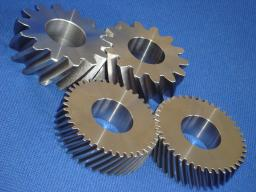Did you find an error or inaccuracy? Feel free to write us. Thank you!

Showing 1 comment:Math student
Is it grammar or do I not understand how ' stained' is used?Tips to related online calculators
Do you want to calculate least common multiple two or more numbers?

## Related math problems and questions:

• Gearing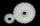Gearing consists of two wheels, one is 88 and second 56 teeth. How many times turns smaller wheel to fit the same teeth as in the beginning? How many times will turn biggest wheel?
• Two gears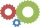Two gears with 13 and 7 teeth rotate locked into each other. How many turns does a big wheel have to make for both wheels to be in the starting position again?
• Clock's gears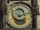In the clock machine, three gears fit together. The largest has 168 teeth, the middle 90 teeth, and the smallest 48 teeth. The middle wheel turns around its axis in 90 seconds. How many times during the day do all the gears meet in the starting position?
• BiketrialKamil was biketrial. Before hill he set the forward gear with 42 teeth and the back with 35 teeth. After how many exercises (rotation) of the front wheel both wheels reach the same position?
• Bicycle wheels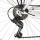The driving wheel of a bicycle has 54 teeth. The driven wheel has 22 teeth. After how many revolutions will meet the same teeth?
• Gcd and lcm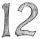Calculate the greatest common divisor and the least common multiple of numbers. a) 16 and 18 b) 24 and 22 c) 45 and 60 d) 36 and 30
• LCMWhat is the least common multiple of 5, 50, 14?
• Two gears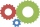The two gears fit together. The larger gear has 32 teeth, the smaller has 20 teeth less. How many times does turn a smaller gear if the bigger gear turns three times?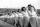Practitioners lined up in rectangle with row with four, five or six exercisers, one always missing to full rectangle. How many exercisers were on the field, if they have estimated not been more than 100?
• Lesson exercising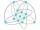The lesson of physical education, pupils are first divided into three groups so that each has the same number. The they redistributed, but into six groups. And again, it was the same number of children in each group. Finally they divided into nine equal g
• Trees in alleyThere are four trees in the alley between which the distances are 35m, 15m and 95m. Trees must be laid in the spaces so that the distance is the same and the maximum. How many trees will they put in and what will be the distance between them?
• Lcm of three numbersWhat is the Lcm of 120 15 and 5
• TilesHow many tiles of 20 cm and 30 cm can build a square if we have a maximum of 100 tiles?
• PlumsIn the bowl are plums. How many would be there if we can divide it equally among 8, 10 and 11 children?
• Lcm simpleFind least common multiple of this two numbers: 140 175.
• Bicycle gears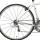The toothed wheel on the bicycle pedal has 40 teeth, the wheel on the rear wheel has only 16 teeth. How many times does the rear wheel turn if the pedals rotate 50 times?
• Tractor wheelsThe front wheel of the tractor has a circumference of 18 dm and the rear 60 dm. We will make a red mark on the lowest point of both wheels. The tractor then starts. At what distance from the start will both marks appear identically at the bottom again?